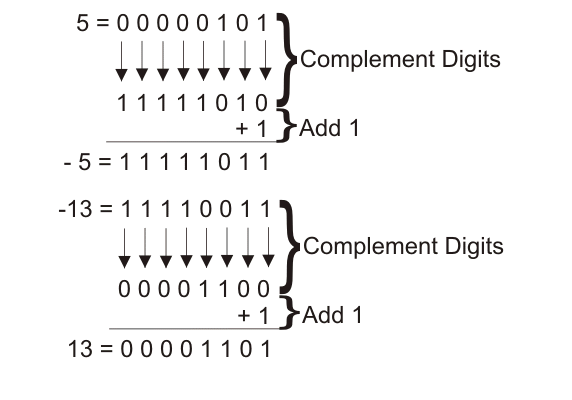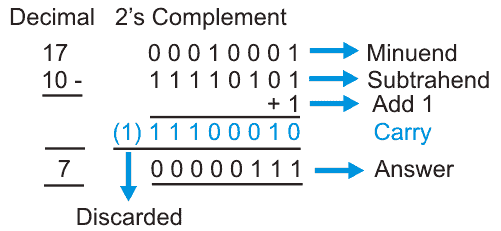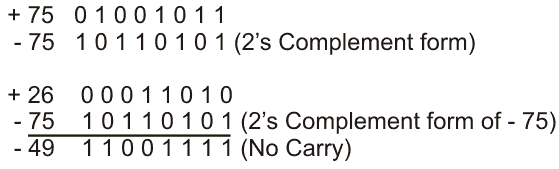# 2′s Complement

### Complement Number System

The word complement in the number system means the difference of the number from the highest number of that digit. This definition can be applied to various number systems and they are named differently as well. But in general, if a number has base of N then that is known as (N-1)’s complement. However, if we add one with that value that will give us N’s complement. For example let us start with decimal number because we are mostly familiar with that number system. Let us take a number 25. The complement can be found out in two ways and since it has a base of 10, which will be called as (10 – 1) that is 9’s complement.
Method 1: The first method is to subtract the number from highest number of that digit which implies number has to be subtracted from 99. So we get (99 – 25) that is 74.

Method 2: In the second method each digit is considered individually and subtracted from 9 since 9 is the highest. So we get 9 – 2, 9 – 5 which means the answer is 74. So we have got the same answer and both the methods of finding complement is true and can be applied according to our will.

Binary Number System: Now coming to a binary number which is our main topic for discussion. It has only two digits 0 and 1 and hence the name is binary. It has a base of 2 so if we subtract it from the highest number of that digit then we get (2 – 1) that is 1’s complement. If 1 is added with that then we will get 2’s complement. Also, if the individual digit is subtracted from 1 (highest among 0 and 1) then also we will get 1’s complement and adding one with that will give us 2’s complement. However, luckily for us there is another method of finding 1’s complement. We do not have to remember all those steps to find out 1’s and 2’s complement but a simple trick will do the job.

Two methods are similar which we have discussed previously but a new method or trick we can use in finding 1’s complement. Let us break the suspense and look at the various methodologies to find out 1’s and 2’s complement with an example. For example, we have to find out 2’s complement of 0100. Here, a zero has been used before the number to make it a four bit number. Since, we have number of bits in the power of two for a binary system.
Method – 1 In this method we have to subtract it from 1111 since it is the highest four digit number to find out 1’s complement. 1111 – 0100 is 1011. 2’s complement will be 1011 + 1 that is 1100.

Method – 2 Here, subtract every individual digit from 1 to get 1’s complement. Whose result will be 1 – 0, 1 – 1, 1 – 0, 1 – 0, 1 – 0 that is 1011.
2’s complement will be 1011 + 1 = 1100.

Method – 3 Here, just we have to replace 1 by 0 and 0 by 1 to find out 1’s complement. Adding one with that result will give us 2’s complement.

For 0100, we will have 1’s complement just by replacing 1 by 0 and 0 by 1 and this will give the result 1011. Adding 1 with that result will give us (1011 + 1) that is 1100.
So in all of the three methods we see that we have found out the same result and all of the methods are correct and one can use any one of them according to its convenience.2’s complement representation of positive and negative number.

### Why We Do Need 2’s Complement?

The main problem of using 2’s complement is that it can be used for subtraction for two binary digits. The computer understands only binary as we know, and there is nothing called as negative number in the binary number system but it is absolutely necessary to represent a negative number using binary which can be done by assigning a sign bit to the number which is an extra bit required. If the sign bit is 1 then number is considered negative and if it is 0, than it will be the number will be called as positive.
For subtraction of binary numbers that can be done as follows-

#### Subtracting a Smaller Number from a Larger Number

1. Find 2’s complement of the smaller number.
2. Add the larger and 2’s complement of the smaller number.
4. After discarding the carry, keep the result which will be answer for subtraction.#### Subtracting Larger Number from Smaller Number

1. Find 2’s complement of larger number.
2. Add 2’s complement of larger number to the smaller number.
3. If no carry is generated, find the 2’s complement of the result and the result will be negative.
4. If carry is generated, discard the carry and take the result which will be the answer and the sign will be negative.Subtraction of larger number from smaller number.

1. Subtraction can be done with the help of 2’s complement method.
2. Easy to accomplish with larger circuit.
3. End around carry need not be performed as in the case of 1’s complement.
4. Negative number can be represented using 2’s complement.

Want To Learn Faster? 🎓
Get electrical articles delivered to your inbox every week.
No credit card required—it’s 100% free.